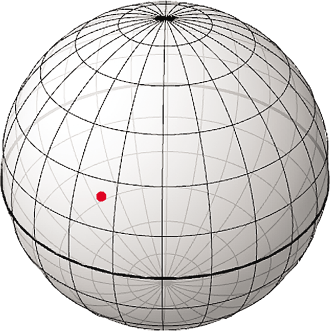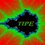# Maximum and minimum value of $2xy+2yz+7xz$This is a problem I proposed for the Final round of the Peruvian Mathematical Olympiad 2013, about 1 month ago. I hope you will enjoy this problem.

Problem Let $x, y, z$ be real numbers such that $x^2+y^2+z^2\leq 100$, find the maximum and minimum values of $2xy+2yz+7xz.$.Note by Jorge Tipe
6 years, 11 months ago

This discussion board is a place to discuss our Daily Challenges and the math and science related to those challenges. Explanations are more than just a solution — they should explain the steps and thinking strategies that you used to obtain the solution. Comments should further the discussion of math and science.

When posting on Brilliant:

• Use the emojis to react to an explanation, whether you're congratulating a job well done , or just really confused .
• Ask specific questions about the challenge or the steps in somebody's explanation. Well-posed questions can add a lot to the discussion, but posting "I don't understand!" doesn't help anyone.
• Try to contribute something new to the discussion, whether it is an extension, generalization or other idea related to the challenge.

MarkdownAppears as
*italics* or _italics_ italics
**bold** or __bold__ bold
- bulleted- list
• bulleted
• list
1. numbered2. list
1. numbered
2. list
Note: you must add a full line of space before and after lists for them to show up correctly
paragraph 1paragraph 2

paragraph 1

paragraph 2

[example link](https://brilliant.org)example link
> This is a quote
This is a quote
    # I indented these lines
# 4 spaces, and now they show
# up as a code block.

print "hello world"
# I indented these lines
# 4 spaces, and now they show
# up as a code block.

print "hello world"
MathAppears as
Remember to wrap math in $$ ... $$ or $ ... $ to ensure proper formatting.
2 \times 3 $2 \times 3$
2^{34} $2^{34}$
a_{i-1} $a_{i-1}$
\frac{2}{3} $\frac{2}{3}$
\sqrt{2} $\sqrt{2}$
\sum_{i=1}^3 $\sum_{i=1}^3$
\sin \theta $\sin \theta$
\boxed{123} $\boxed{123}$

Sort by:

I hate to do this, but I feel inclined to bash using Lagrange multipliers. For any $x^2+y^2+z^2=k$, $0\le k\le100$, we want $\left<2y+7z,2x+2z,2y+7x\right>=\lambda\left<2x,2y,2z\right>$. Solving for $\lambda$, we get $2y+7z=2x\lambda$, $2x+2z=2y\lambda$, and $2y+7x=2z\lambda$. Eliminate $x$ by substituting $x=y\lambda-z$ to get $2y+7z=2y\lambda^2-2z\lambda$ and $2y+7(y\lambda-z)=2z\lambda$. Plugging in the second as $2z\lambda$, we get the quadratic $0=2y\lambda^2-7y\lambda-4y=y(2\lambda+1)(\lambda-4)$, so $\lambda=-\frac{1}{2}\text{ or }4$, or $y=0$. Whew, $\lambda$ is somewhat easy to work with (thanks Jorge!).

In the case that $y=0$, we have $7xz$ to optimize. Assume WLOG that $x,z\ge0$, so $7xz\le7\left(\sqrt{\frac{x^2+z^2}{2}}\right)^2\le7\frac{100}{2}=350$. That means $y=0$ gives a maximum of $350$ ($x=z=5\sqrt{2}$) and minimum of $-350$ ($x=5\sqrt{2},z=-5\sqrt{2}$).

In the case that $\lambda=4$, $2y+7z=4(2x)$ and $2x+2z=4(2y)$. Solving for $y$ and $z$, $y=\frac{1}{2}x$ and $z=x$, which plugs in as $2xy+2yz+5zx=9x^2$ and $x^2+y^2+z^2=\frac{9}{4}x^2\le100$. The minimum here is $0$ ($x=y=z=0$) clearly, but the maximum is $9\frac{4}{9}100=400$ ($x=z=\frac{20}{3},y=\frac{10}{3}$).

In the case that $\lambda=-\frac{1}{2}$, $2y+7z=-\frac{1}{2}(2x)$ and $2x+2z=-\frac{1}{2}(2y)$. Solving for $y$ and $z$, we get $y=-4x$ and $z=x$, which plugs in as $2xy+2yz+5zx=-11x^2$ and $x^2+y^2+z^2=18x^2\le100$. The maximum here is clearly $0$ ($x=y=z=0$), but the minimum is $-4\frac{100}{18}=-\frac{550}{9}$ ($x=z=\frac{5\sqrt{2}}{3},y=-\frac{20\sqrt{2}}{3}$).

In effect, the maximum value is $\boxed{400}$ at $\left(\frac{20}{3},\frac{10}{3},\frac{20}{3}\right)$, and the minimum value is $\boxed{-350}$ at $\left(5\sqrt{2},0,-5\sqrt{2}\right)$.

Now the real question is what is the non-LM solution?

- 6 years, 11 months ago

Of course there is a elementary solution, in fact this problem was proposed to students with no knowledge of Calculus (grades 9 and 10 in Peru) and only one student managed to solve both parts of the problem.

- 6 years, 11 months ago

Is it Jensen's?

- 6 years, 11 months ago

This is exactly how I would've done it, great solution, regardless of any bashing

- 6 years, 3 months ago

If we want the greatest possible value of $2xy+2yz+2xz$ (instead of $2xy+2yz+7xz$ ) we can use the inequalities $2xy\leq x^2+y^2$, $2yz\leq y^2+z^2$ and $2xz\leq x^2+z^2$, in this case the maximum occurs when $x=y=z$.

But in our problem we can try to use similar inequalities like $2xy\leq Ax^2+By^2$, where $A$ and $B$ are suitable constants.

- 6 years, 11 months ago

For minimum: $2xy+2yz+7xz = (x+y+z)^2 - (x^2+y^2+z^2) + \frac{5}{2} (x+z)^2 - \frac{5}{2}(x ^2 + z^2) \\ \ge - (x^2+y^2+z^2) - \frac{5}{2}(x ^2 + z^2) \ge -100 - \frac{5}{2} (100) = -350.$ The equality holds when $x+y+z=0, x+z=0, x^2+y^2+z^2=100, x^2+z^2=100$. There is an obvious solution, that is $y=0, x=-z, x^2=z^2=50$.

- 6 years, 11 months ago

For me is very nice to see new solutions of this problem. Let me show my approach:

First note that $x$ and $z$ have a symmetric role. Then, to obtain $x^2+y^2+z^2$ I will work with a expression of the form $(x+\lambda y+z)^2$ [note that $x$ and $z$ have the same coefficient]]. If we want a multiple of $2xy+2yz+7xz$ we can take $\lambda=\frac{2}{7}$.

Thus we work with the following inequality which is true: $\left(x+\frac{2}{7}y+z\right)^2+\frac{45}{49}y^2 \geq 0,$ this inequality is equivalent to: $\frac{2}{7}(2xy+2yz+7xz)\geq -x^2-y^2-z^2$ and we are done! [Of course, we have to complete some details]

- 6 years, 11 months ago

Very nice, interesting approach.

- 6 years, 11 months ago

What was your motivation behind breaking it up like this?....

- 6 years, 10 months ago

Really nice approach.

- 6 years, 10 months ago

2xy+2yz+7xz=what?

- 6 years, 11 months ago

Let $k = 2xy+2yz+ 7zx$. Then $k \leq \dfrac{100( 2xy+2yz+ 7zx)}{x^2+y^2+z^2}$, which becomes \begin{aligned} kx^2-x(200y+700z)+ky^2-200yz+kz^2\leq 0. \end{aligned} Since the solutions of $kx^2-x(200y+700z)+ky^2-200yz+kz^2 = 0$ are real (solutions are $x$ and $\dfrac{200y+700z}{k}-x$), its discriminant $\Delta_x$ is nonnegative: \begin{aligned} \Delta_x = (200y+700z)^2-4k(ky^2-200yz+kz^2) &\geq 0\\ \implies (40000-4k^2)y^2 + (280000+800k)yz+(490000-4k^2)z^2&\geq 0.\qquad(\star) \end{aligned} If $z = 0$, then $(\star)$ becomes $(40000-4k^2)y^2 \geq 0$ and since $y^2\geq 0$ for all $y\in\mathbb{R}$ we must have $40000-4k^2\geq 0\implies |k|\leq 100$. If $z\neq 0$, then dividing both sides of $(\star)$ and taking $t=\dfrac{y}{z}$ gives \begin{aligned} (40000-4k^2)t^2 + (280000+800k)t+(490000-4k^2)\geq 0. \end{aligned} Since the roots of the equation $(40000-4k^2)t^2 + (280000+800k)t+(490000-4k^2)=0$ are real (note that $t = \dfrac{y}{z}\in\mathbb{R}$, its discriminant $\Delta_t$ is nonnegative: \begin{aligned} \Delta_t = (280000+800k)^2-4(40000-4k^2)(490000-4k^2)\geq 0\\ \implies k^3-142500k-7000000\leq 0. \end{aligned} (I used Wolfram Alpha to obtain this cubic inequality). Since the roots of $k^3-142500k-7000000=0$ are $k=-350, -50, 400$ (also obtained from Wolfram Alpha), the minimum value of $k = 2xy+2yz+7zx$ is $\boxed{-350}$ at $(x,y,z)=(-5\sqrt{2}, 0, 5\sqrt{2})$ and the maximum value is $\boxed{400}$ at $(x,y,z)=\left(\dfrac{20}{3}, \dfrac{10}{3}, \dfrac{20}{3}\right)$.

- 6 years, 3 months ago

Woah! Amazing solution! Truly genius, in my opinion!

- 6 years, 3 months ago

For maximum: $2xy+2yz+7xz = 4(x^2+y^2+z^2) - \frac{1}{2}((x-2y)^2+(z-2y)^2 + 7(x-z)^2) \le 400.$ The equality holds when $x=2y=z$ and $x^2+y^2+z^2=100$.

Or apply Cauchy-Schwarz to:

$(2xy+2yz+7xz)^2 = [(x)(2y) + (2y)(z) + (x)(z) + (x)(z)+ (x)(z) + (z)(x) + (z)(x)+(z)(x)+(z)(x)]^2\\ \le[x^2+(2y)^2+x^2+x^2+x^2+z^2+z^2+z^2+z^2][(2y)^2+z^2+z^2+z^2+z^2+x^2+x^2+x^2+x^2]\\ =(4x^2+4y^2+4z^2)^2$

Or simply as Jorge pointed out: $2xy = x(2y)\le \frac{1}{2}(x^2+4y^2), 2yz = (2y)z\le \frac{1}{2}(z^2+4y^2), 7xz \le \frac{7}{2}(x^2+z^2)$ then add all three together.

- 6 years, 11 months ago

Why has the diagram of a sphere been given?

- 6 years, 11 months ago

It's only a point inside a sphere :) If $x^2+y^2+z^2\leq 100$ then the point $(x,y,z)$ is inside the sphere of radius 10.

- 6 years, 11 months ago

I have no idea what to do here.I tried establishing a bound but I am not sure what to use here.I have tried to use some elementary inequalities such as Cauchy-Schwarz and AM-GM.Using AM-GM,we find that

$\dfrac{x^2+y^2+z^2}{3}\ge [(xy)(yz)(zx)]^\dfrac{1}{3}\le \dfrac{xy+yz+zx}{3}$

But I am not sure how that helps us.Also,

$x^2+y^2+z^2\ge \dfrac{(x+y+z)^2}{3}$

by Titu's lemma.I have also tried to rewrite the expression as

$2xy+2yz+7zx=2(xy+yz+zx)+5zx$

and was wondering whether I can apply AM-GM here.

- 6 years, 11 months ago

I think something like $2xy+2yz+7zx\le (2^2+2^2+7^2)(x^2y^2+y^2z^2+z^2x^2)=57(x^2y^2+y^2z^2+z^2x^2)\le 57(x^4+y^4+z^4)$ would help, because $x^2y^2$ (and similar terms) are nonnegative.

- 6 years, 11 months ago

That gives you an upper bound on the possible value of $2xy+2yz+7zx$. Another (easy) upper bound is that since $|x|, |y|, |z| \leq 10$, hence the value is at most $200+200+700=1100$. Hence, we also need to check and see if it is indeed the least upper bound.

For it to be the least upper bound, we must have equality hold throughout. What are the equality conditions for each of your inequalities?

Staff - 6 years, 11 months ago

Hi Rahul! If I'm not mistaken for using AM. G.M the numbers on which the inequality is applied must be positive, here is there any condition that $x,y,z$ is positive?

- 6 years, 11 months ago

No,but $x^2,y^2,z^2$ are always non-negative.However,when I say

$[(xy)(yz)(zx)]^\dfrac{1}{3}\le \dfrac{xy+yz+zx}{3}$

I am possibly wrong,since we don't know if $xy,yz,zx$ are non-negative.However,when looking for the maximum,we can safely assume that $x,y,z$ are positive.

NOTE: AM-GM stipulates the values being non-negative ,not positive.

- 6 years, 11 months ago

I see! Thanks Rahul!

- 6 years, 10 months ago

how can i become as intelligent as you

- 6 years, 11 months ago

Definitely not by asking "how can i become as intelligent as you."

- 6 years, 11 months ago

If you think being sarcastic sounds clever, you're highly mistaken.

- 6 years, 11 months ago

The tone of sarcasm rarely transmits well over the internet, so let's avoid it. I'm sure we can come up with several pointers, like:

Practice, practice, practice
Learn from others

Staff - 6 years, 11 months ago

How can you be so rude??

- 6 years, 2 months ago